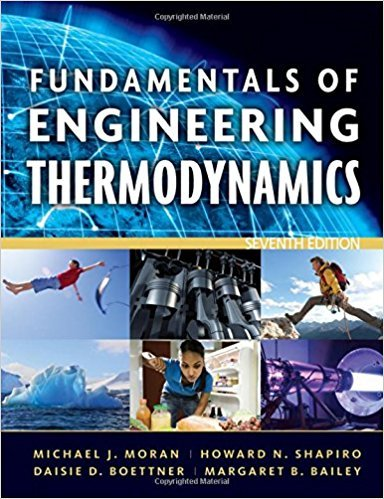×
Get Full Access to Fundamentals Of Engineering Thermodynamics - 7 Edition - Chapter 1 - Problem 30p
Get Full Access to Fundamentals Of Engineering Thermodynamics - 7 Edition - Chapter 1 - Problem 30p

×

# Using Specific Volume, Volume, and PressureFigure P1.30ISBN: 9780470495902 50

## Solution for problem 30P Chapter 1

Fundamentals of Engineering Thermodynamics | 7th Edition

• Textbook Solutions
• 2901 Step-by-step solutions solved by professors and subject experts
• Get 24/7 help from StudySoup virtual teaching assistantsFundamentals of Engineering Thermodynamics | 7th Edition

4 5 1 419 Reviews
22
4
Problem 30P

Problem 30P

Using Specific Volume, Volume, and Pressure

Figure P1.30 shows a gas contained in a vertical piston-cylinder assembly. A vertical shaft whose cross-sectional area is 0.8 cm2 is attached to the top of the piston. Determine the magnitude, F, of the force acting on the shaft, in N, required if the gas pressure is 3 bar. The masses of the piston and attached shaft are 24.5 kg and 0.5 kg, respectively. The piston diameter is 10 cm. The local atmospheric pressure is 1 bar. The piston moves smoothly in the cylinder and g = 9.81 m/s2.Fig. P1.30

Step-by-Step Solution:

Step 1 of 3:

The area of the vertical shaft attached to the shaft is given. The force applied on the container depends on the pressure inside the container, the atmospheric pressure and the weight of the piston plus shaft. We are going to find the magnitude of the force. The piston experiences zero friction on the walls of the container.

The area of cross-section of the shaft A = 0.8 cm2 = 8 x 10-5 m2

The combined mass of the shaft and the piston m = 24.5 kg + 0.5 kg

The diameter of the piston d = 10 cm = 0.1 m (r = 0.05 m)

The atmospheric pressure pa = 1 bar = 105 Pa

The pressure inside the container p = 3 bar = 3 x 105 Pa

The acceleration due to gravity g = 9.81 m/s2

Step 2 of 3

Step 3 of 3

##### ISBN: 9780470495902

Unlock Textbook Solution